Physics - Electromagnetism - Mutual and Self Induction exercises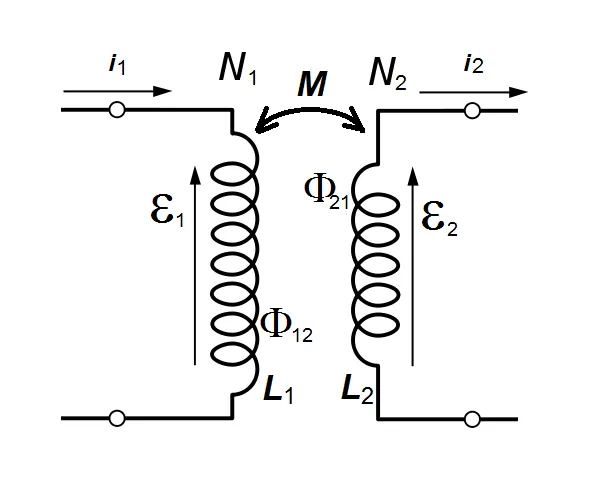Introduction

Hello it's a me again Drifter Programming! Today we continue with Electromagnetism to get into Exercises/Examples all around the Mutual and Self Induction "chapter" we covered in the previous articles. By that I mean examples around Mutual Inductance, Self Induction, Magnetic Energy Density, R-L circuits and L-C circuits. R-L-C circuits have been covered mostly theoretically and so no mathematical applications will be covered yet. So, without further do, let's get started!

To stay organized each article/topic will be covered on it's own...as always!

Mutual Inductance

First up the Mutual Inductance and induced emf between two conductors (we covered coils on N turns that are more interesting).

Example 1:

Let's consider two insulated wires that are wound into a common cylinderical former of l=0.1m length. The cross-sectional area is A = 0.05m^2. The first wire has N1 = 100 turns, whilst the second one has N2 = 300 turns. Let's calculate:

1. The Mutual inductance (M) of the two wires
2. If a current I1 is flowing in the first wire and increases from 0 to 10A in 0.1s, what will be the emf generated on the second wire? Will the produced emf/current act in the same or opposite sense?

1.

As we know from the corresponding article, Mutual inductance is a purely geometrical quantity which doesn't depend on the current and is given by: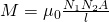For our example the Mutual inductance of the two wires is therefore: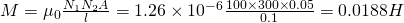2.

The induced emf generated around the second loop can be calculated by: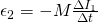The negative sign already shows us that the current on the second wire circulates in opposite sense. According to Lenz's law: "The induced emf/current always tries to oppose the change in magnetic flux that caused it!".

By entering the values of each quantity we can calculate the actual emf, which is: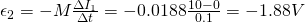If the resistance of the second wire was also given to us, then the induced current could be calculated using Ohm's law as: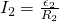Example 2:

Let's now consider two identical coils that are wound on a ring-shaped iron core with relative permeabilty μr = 500. Each coil has 100 turns and the cross-sectional area is A = 3cm^2. The magnetic path length is l = 20cm. Calculate the Mutual Inductance between the coils by calculating the inductance of each coil and then using: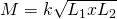This example contains Mutual Inductance and Self Induction!

The inductance of each coil is also a purely geometrical quantity. As we know from the article of the series this inductance is calculated as: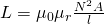For the values of our example the inductance of each coil is therefore: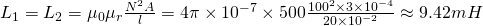Using the given equation the Mutual inductance is:Note: Being wound on the same iron core means that k = 1.

Self Induction

Continuing on with Self Inductance...

Example 1:

Let's consider a solenoid of N = 900 turns has a total magnetic flux of Φ = 1.33 x 10^-7Wb through its air core (no relative permeability required here) when the coil current is 100mA. If the flux takes up t = 75ms to grow from zero to max, calculate:

1. the Inductance of the coil
2. the counter-emf (self-induced emf) that is being created during that flux growth

1.

Another way of calculating the Inductance of a coil is using the rate of magnetic flux per current change (dΦ/di) times the number N of coils. Using this equation the Inductance is: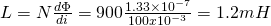2.

The self-induced emf in the coil caused by the magnetic flux change is: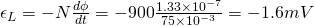The negative sign shows us that the induced emf acts in the opposite sense!

Example 2:

Let's now also consider a hollow air-corded inductor coil of N = 500 turns of wire which produces a magnetic flux of Φ = 10mWb when a DC current of I = 10A passes through it. Calculate:

1. the Self-Inductance of the coil
2. The self-induced emf after a time of t = 10ms

1.

The Self-Inductance is simply: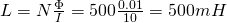2.

Therefore the self-induced emf is: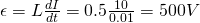A very simple example, just to show you that only the "max" value matters!

Magnetic (and Electric) Energy Density

Let's now get into a somehow different topic, which has to do with magnetic and electric energy and more specifically the density of it...

Example 1:

Let's consider a region of space with a magnetic field of T = 1 x 10^-2T and a electric field of E = 2x10^6 V/m. Calculate the total/combined energy density of the electric and magnetic fields.

The energy density of the electric field can be calculated using: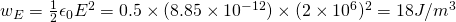The magnetic energy density is: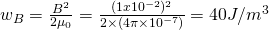The net energy density is the sum of each energy and so: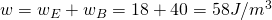Example 2:

Another complete, solved (and almost identical) example can be found at:

R-L circuits

Let's now get into circuits, starting off with R-L (or L-R) circuits!

Example 1:

Let's consider a coil with inducatance L = 40mH and resistance R = 2Ω that is connected in series with a V = 20V DC power supply and "separated" by a switch. Let's calculate the:

• time constant (τ)
• transient time
• induced emf (ε) after 10ms
• current after one time constant (after closing a switch).

a)

The maximum current that can flow through a LR series circuit is known as steady state current and can be calculated using Ohm's law as: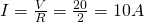b)

The time constant (τ) in a RL series circuit can be calculated by: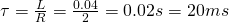c)

The transient time for RL series circuit is approximately equal to 5 times the transient time and so:d)

Using the equation that we covered in the series/article we can find the emf/voltage after 10ms being: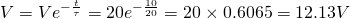e)

The current after t = τ can be found using the corresponding equation as: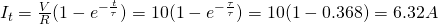Example 2:

In this example we will now calculate the current without using the equations, by using a table of percentage that can be found at:

Let's consider a simple R-L circuit with an inductance of L = 1H, resistance of R = 5Ω and energy source of V = 10V.

Let's calculate the:

1. maximum / steady state current
2. time constant (τ)
3. current after t = 0.5 τ
4. current after t = τ
5. current after t= 2τ

1.

Using Ohm's law we have:2.

The time constant is: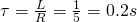3.

The current after 0.5τ will have reached 40% of it's final/ultimate value (by taking a look on the table) and so is: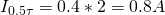4.

Using the table we see that the current will reach 63.2% of it's maximum value after τ seconds.

That way: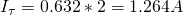5.

After t = 2τ the curent will reach 86.5% of it's final value which is:Note: After t = 5τ the current is not exactly I, but 99.3% of the max value!

L-C circuits

Let's now also get into a L-C circuit...Yeh, only one :P

Consider an oscillating LC circuit made of an 2μH inductor and 10nF capacitor. The maximum charge of the  capacitor is 0.4μC. Let's find:

• The total energy of the circuit
• The absolute values of charge in the capacitor and current in the inductor the instant when 1/4 of the total energy is electric energy in the capacitor.
Such circuits are very easy to play with, cause everything has to do with energies...

a)

Because of the conservation of energy and cause no energy is being lost as heat in a resistor, the total energy in the circuit stays the same and can be found at any instant of the oscillation. This means that the total energy can be found using the maximum charge of the capacitor, maximum current in the inductor, or even as a sum of the individual energies.

The maximum energy using the maximum charge of the capacitor is:b)

Knowing that the energy remains constant the magnetic energy will be 3/4 of the total energy, cause 1/4 is already being stored in the capacitor.

Therefore: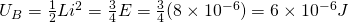Knowing the inductance, the current flowing in the circuit/inductor (in series circuits the current is the same for all the components) can be found easily now as:The absolute value of the capacitor charge can be calculated similarly as:Any case can be calculated in a similar way!

REFERENCES:

Mathematical equations that I had in this post where drawn using quicklatex!

Electric fields:

Getting into Electromagnetism -> electromagnetim, electric charge, conductors, insulators, quantization

Coulomb's law with examples -> Coulomb's law, superposition principle, Coulomb constant, how to solve problems, examples

Electric fields and field lines -> Electric fields, Solving problems around Electric fields and field lines

Electric dipoles -> Electric dipole, torque, potential and field

Electric charge and field Exercises -> examples in electric charges and fields

Electric flux:

Electric flux and Gauss's law -> Electric flux, Gauss's law

Applications of Gauss's law (part 1) -> applying Gauss's law, Gauss applications

Applications of Gauss's law (part 2) -> more Gauss applications

Electric flux exercises -> examples in electric flux and Gauss's law

Electric potential:

Electric potential energy -> explanation of work-energy, electric potential energy

Calculating electric potentials -> more stuff about potential energy, potential, calculating potentials

Millikan's Oil Drop Experiment -> Millikan's experiment, electronvolt

Cathode ray tubes explained using electric potential -> cathode ray tube explanation

Electric potential exercises (part 1) -> applications of potential

Electric potential exercises (part 2) -> applications of potential gradient, advanced examples

Capacitance:

Capacitors (Condensers) and Capacitance -> Capacitors, capacitance, calculating capacitance

How to solve problems around Capacitors -> combination, solving problems, simple example

Electric field energy and density -> Electric field energy, energy density

Dielectric materials -> Dielectrics, dielectric constant, permittivity and strength, how to solve problems

Electric capacitance exercises -> examples in capacitance, energy density and dielectrics

Current, resistance and EMF:

Electric current -> Electric current, current density

Electrical resistivity and conductivity -> Electrical resistivity, conductivity, thermal coefficient of resistivity, hyperconductivity

Electric resistance -> Resistance, temperature, resistors

Electromotive Force (EMF) and Internal resistance -> Electromotive force, internal resistance

Power and Wattage of Electronic Circuits -> Power in general, power/wattage of electronic circuits

Electric current, resistance and emf exercises -> exampes in all those topics

Direct current (DC) circuits:

Resistor Combinations -> Resistor combinations, how to solve problems

Kirchhoff's laws with applications -> Kirchhoff's laws, how to solve problems, applications

Electrical measuring instruments -> what are they?, types list, getting into some of them, an application

Electronic circuits with resistors and capacitors (R-C) -> R-C Circuit, charging, time constant, discharging, how to apply

RC circuit exercises -> examples in Kirchhoff, charging, discharging capacitor with/without internal resistance

Magnetic field and forces:

Magnetic fields -> Magnetism, Magnetic field

Magnetic field lines and Gauss's law of Magnetism -> magnetic field lines, mono- and dipoles, Flux, Gauss's law of magnetism

The motion of charged particles inside of a magnetic field -> straight-line, spiral and helical particle motion

Applications of charged particle motion -> CERN, Cyclotrons, Synchrotrons, Cavity Magetron, Mass Spectrometry and Magnetic lens

Magnetic force applied on Current-Carrying Conductors -> magnetic force on current-carrying conductors/wires, proofs

Magnetic force and torque applied on current loops (circuits) -> magnetic force on current loops, magnetic moment and torque

Explaining the Physics behind Electromotors -> tesla, history and explaining the physics behind them

Magnetic field exercises -> examples in magnetic force, magnetic flux, particle motion and forces/torque on current-carrying conductors

Magnetic field sources:

Magnetic field of a moving charged particle -> moving charge, magnetic field, force between parallel charged particles

Magnetic field of current-carrying conductors -> magnetic field of current, Biot-Savart law

Force between parallel conductors and the magnetic field of a current loop-> force between parallel conductors, magnetic field of current loop

Ampere's law and Applications -> Ampere's law, applications

Magnetic materials -> Magnetic materials, classification and types, material examples

Displacement current -> Displacement current, Extension of Ampere's law

Exercises in Magnetic field sources -> examples all around magnetic field sources

Electromagnetic Induction:

Motional Electromotive Force (Emf) -> Motional Emf, Faraday's law and motional emf, generalization

Lenz's law and Induced Electric fields -> Lenz's law, Induced Electric Fields

Eddy Currents and Applications -> Εddy currents, applications (brakes, testing, others)

Maxwell's equations -> What they are, each equation analyzed separately

Electromagnetic Induction exercises -> examples all around Electromagnetic Induction

Mutual and Self Induction:

Mutual Inductance -> Mutual Inductance, applications

Self Induction -> Self Induction, Lenz's law, Inductance of a Coil

Magnetic Energy Density -> Energy stored in a magnetic field (or inductor), Magnetic energy density, Coaxial Cable Inductance Example

R-L circuits -> R-L circuit energizing, de-energizing, Characteristic time constant

L-C circuits -> L-C circuit, oscillations, energy cases, applications

R-L-C circuits -> R-L-C circuit, oscillation, applications

And this is actually it for today's post and I hope that you learned how to apply all the things that we covered in actual mathematical problems! After my vacation we will continue with Alternating current :) Yeh, I know I'm in vacation all the Summer. Next up: "Limnos". Articles about it will start by Monday ;)

Bye bye!

H2
H3
H4
3 columns
2 columns
1 column